## 1. Introduction

In this tutorial, we’ll be discovering how to reconstruct a tree from its depth-first traversals. We’ll be using a binary tree as an example to demonstrate and we’ll find out which traversal combinations can be used together to recreate a unique tree.

## 2. Traversals Which Can Be Used to Reconstruct a Tree

As we know, binary trees can be represented by different types of traversals. These traversals can be used to reconstruct a tree. However, usually, one type of traversal is not enough to reconstruct a tree, and we must use two traversals in combination.

The following are the types of depth-first traversals which we can use in combination to reconstruct a tree:

• In-order and pre-order
• In-order and post-order
• Pre-order and post-order – can be used only if the tree is a full binary tree.

As we can see, the in-order traversal is always essential to be able to get a unique tree, unless a binary tree is a full binary tree. In such a case, we can actually reconstruct it from it pre-order and post-order traversal. We’ll learn more about this later in this tutorial.

## 3. A Practical Example

We’ll be using the following tree as our example – we’ll try to reconstruct it using different traversals methods: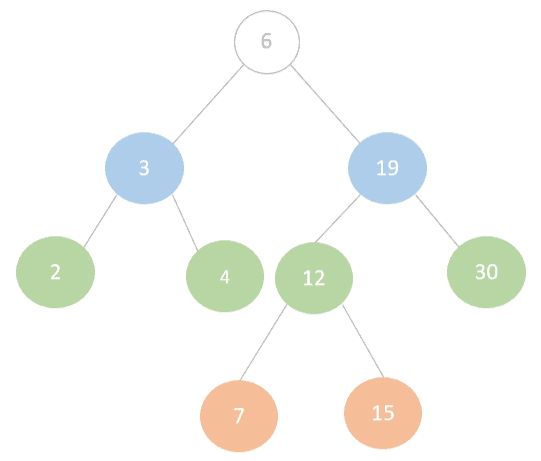Using the different traversal methods on this tree, we get the following traversal sequences: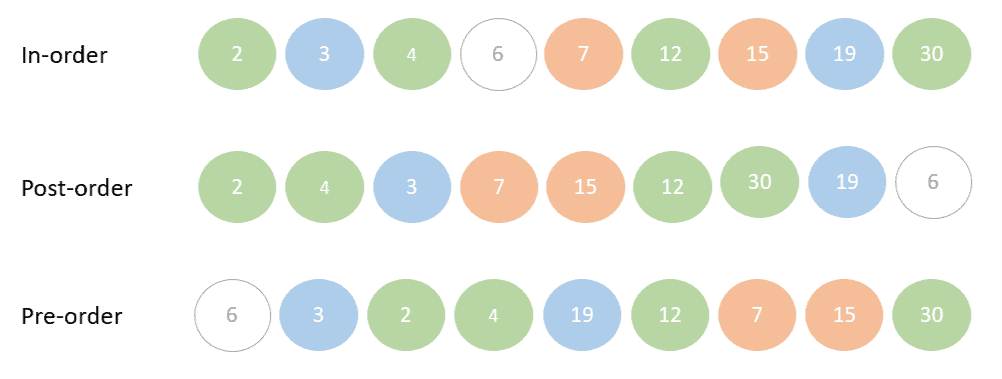## 4. Reconstructing a Tree From Its Pre-Order and In-Order

To reconstruct our tree from its pre-order and in-order sequences, we start by looking at the first element of the pre-order sequence. Since pre-order traverses a tree starting with the root, then the left node, then the right node, we know that the first element 6 is the root of our tree.

Next, we look at the in-order sequence and locate the root 6. Any elements in the in-order sequence to the left of 6 belong to the left sub-tree. Whereas any elements to the right of 6 belong to its right sub-tree:So now we can start building our tree partially to look like this: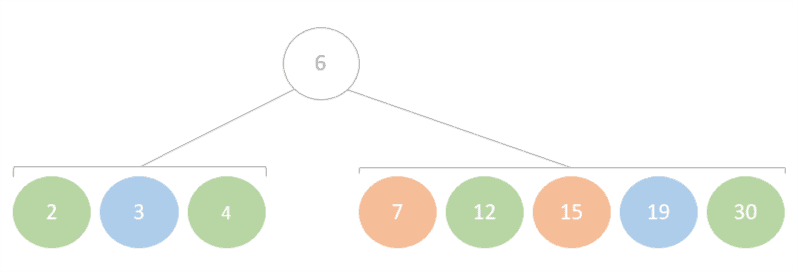Next, we go back to the pre-order sequence and take the next element – which is 3. We already know from our previous step that 3 belongs in the left sub-tree. If we find 3 in the in-order sequence we’ll find that it has 2 and 4 to its left and right sub-tree respectively. i.e. 3 is the root node of the left sub-tree and 2 and 4 are left and right nodes of 3: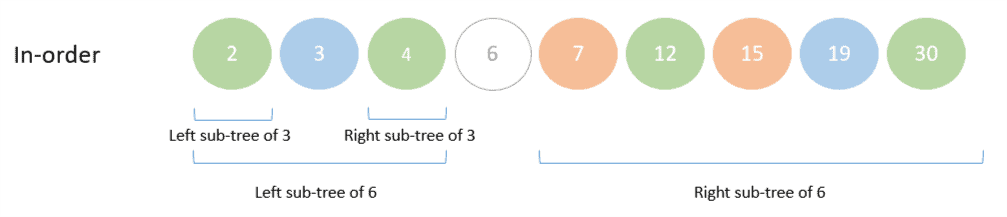Now our tree is shaping up nicely with the left side of the tree entirely built: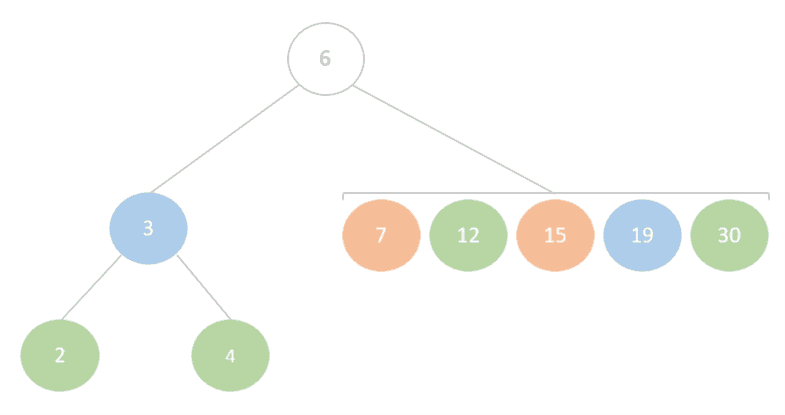Next in the pre-order sequence is 19, which if we find in the in-order sequence gives us the following tree: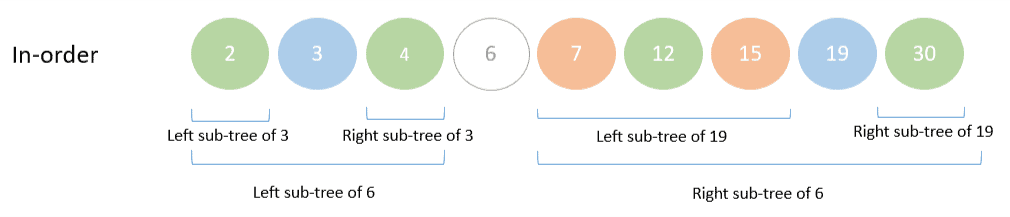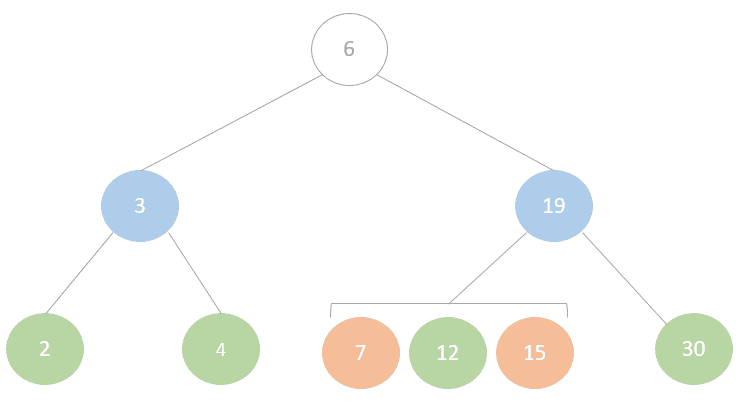As we can see from the previous steps, there’s a recursive pattern emerging in which we traverse the pre-order sequence and for each node position within the in-order sequence and use it to determine its left and right children.

Finally, the next number in the pre-order is 12, and looking at the in-order sequence tells us that nodes 7 and 15 are its left and right children respectively which gives us the final representation of the tree:### 4.1. Algorithm for Reconstructing a Tree From Its Pre-Order and In-Order

Let’s take a look at the following algorithm which describes the logic in pseudo-code. Note, that we’re assuming the following in the algorithm:

• Nodes are a construct with references to left and right child nodes
• indexOf() is a method used to find the index of an element in an array
• length will give us the length of an array## 5. Reconstructing a Tree From Its Post-Order and In-Order

Reconstructing a tree from a post-order and in-order sequence is very similar to reconstructing it using the pre-order and in-order sequence. However, in this case, we’re going to start by looking at the last element in the post-order sequence. Since a post-order sequence always ends with the root node, we know the last element 6 has to be the root of our tree.

Similar to our previous example, in the next step we find node 6 in the in-order sequence and find out the node which belongs to its left and right children.

We’ll continue along the post-order sequence from its last element and repeating these steps until we’ve built up the entire tree: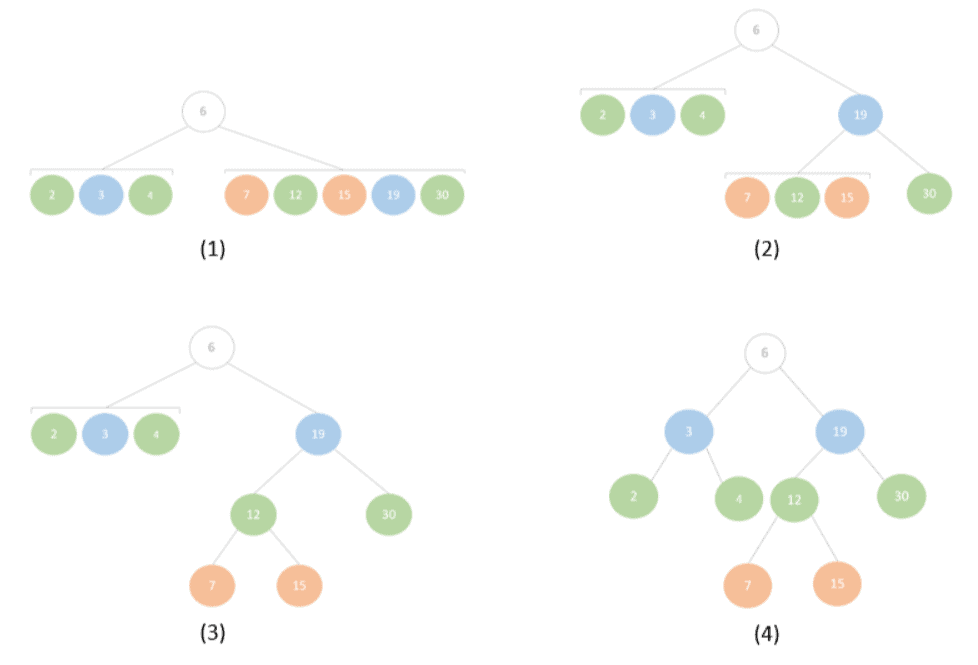### 5.1. Algorithm for Reconstructing a Tree From Its Post-Order and In-Order

The pseudo-code for reconstructing a tree from its post-order and in-order sequences is very similar to our previous pseudo-code example with the difference being in the fact that we start traversing the post-order sequence from back to front and we also start populating the right tree first. This is in keeping with the fact that the post-order traversal traveled backward will give us root, left, and then right nodes. This gives us the following:## 6. Reconstructing a Tree From Its Post-Order and Pre-Order

Lastly, we’re going to see how we can reconstruct a tree from its pre-order and post-order sequence. In order for us to be able to reconstruct a tree from its pre-order and post-order our tree must be a full binary tree. That means each node in the tree must have 0 or 2 nodes.

If you’re wondering why these two sequences can’t be used for binary trees in general, let’s take a look at the two binary trees below which are not full: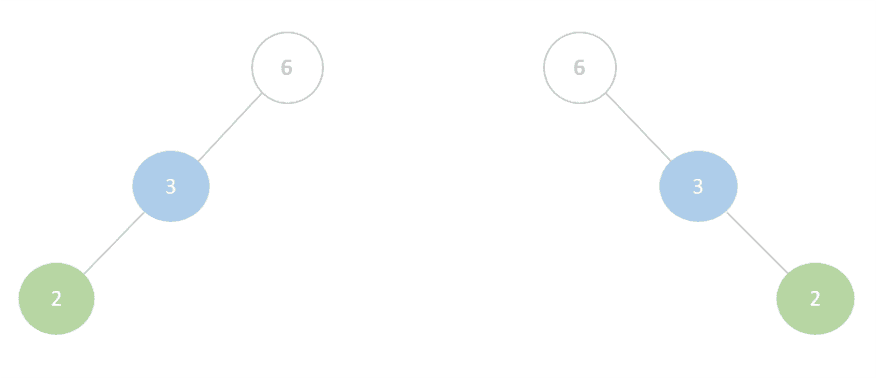Since these two trees have an identical pre-order and post-order sequence, it’s not possible for us to reconstruct each tree individually from those two sequences alone because the pre-order and post-order sequences can’t tell us if a node is a left or a right child.

Because our example tree is actually a full binary tree, we can, in fact, reconstruct it from its pre-order and post-order alone.

We start by determining the node from the first element of the pre-order sequence. Then because we know that we’re dealing with a full binary tree, we can deduce that the next element 3 in the pre-order sequence is surely the left child of the root node.

Now, if we find 3 in the post-order sequence we can use its position to determine which elements are children of 3:Using this information, we get the following partial tree: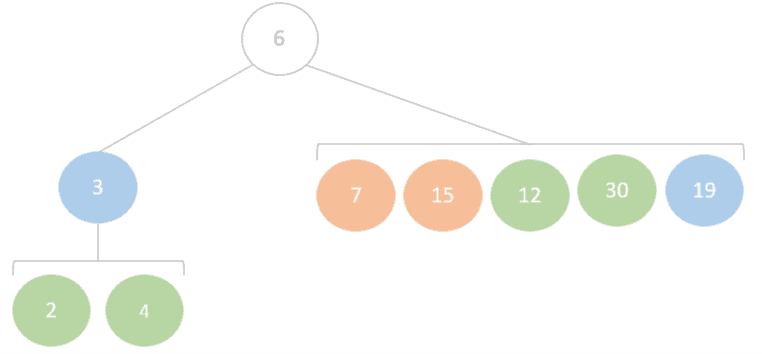The next element after 3 in the pre-order is 2. knowing that this is a full binary tree we can conclude that 2 is the left child of 3 which leaves 4 to be the right child of 3: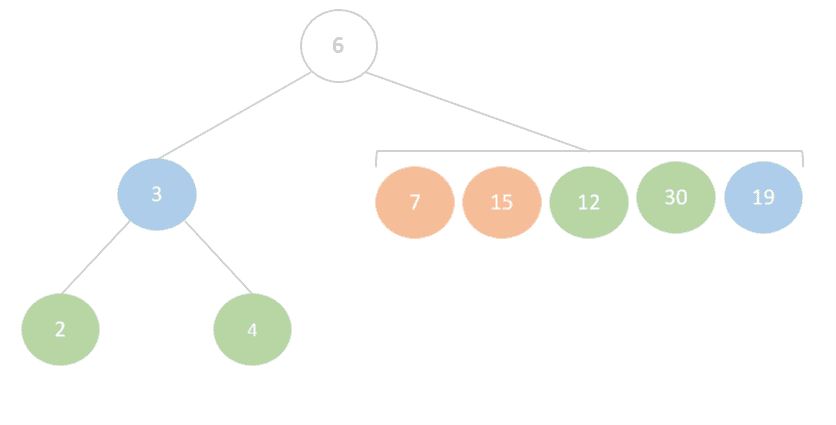Next in the pre-order sequence is number 19, whose position in the post-order sequence shows as the root of the left sub-tree: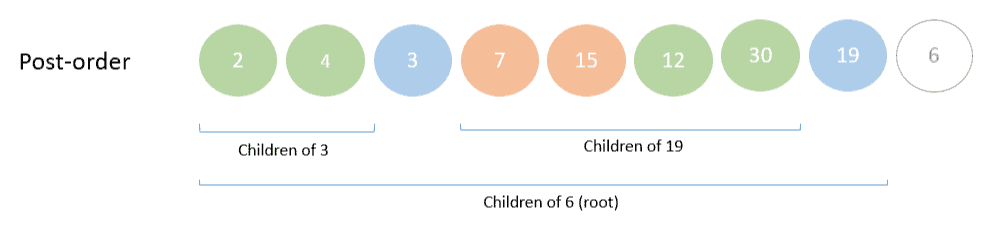Based on this, we can rearrange our tree to give us the following: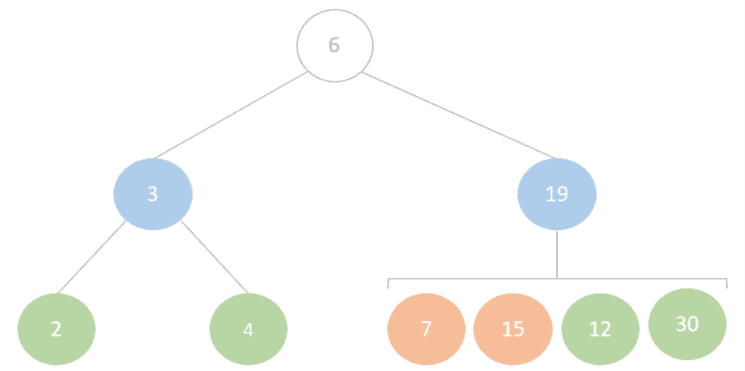Once again, here we can see a recursive pattern which we’re using to build the tree in iterations. First, we use the pre-order sequence to go through each node. Then we use the post-order sequence to find the children of each node. The fact that the binary tree is full helps us determine which node is the left child from the pre-order sequence.

### 6.1. Algorithm for Reconstructing a Tree From Its Post-Order and Pre-Order

Now lets see how we can describes the algorithm with which we can recursively construct a tree from its pre-order and post-order sequences:## 7. Summary

In this tutorial, we discovered various ways of reconstructing a binary tree from its depth-first traversals. In addition, we implemented our knowledge on an example tree and learned the limitation of using pre-order and post-order traversal only to reconstruct a tree.